# 8.2.1 Air emissions calculations

Emission calculation of an individual shipment or for a functional unit of shipment is carried out in the following two steps:

1. Calculation of the total emission for the flight
2. Allocation of the shipment or functional unit based on the weight and the total weight of all cargo

These steps are repeated for every step of the transport chain.

To be able to perform the above steps certain information is needed such as the distance of each flight, the vehicle used (i.e. aircraft model), the load factor and of course the weight of the shipment or the functional unit chosen.

Since air cargo is loaded shipment by shipment or in special air cargo containers or pallets an appropriate weight of this “secondary packaging” should be included in the total weight of shipments.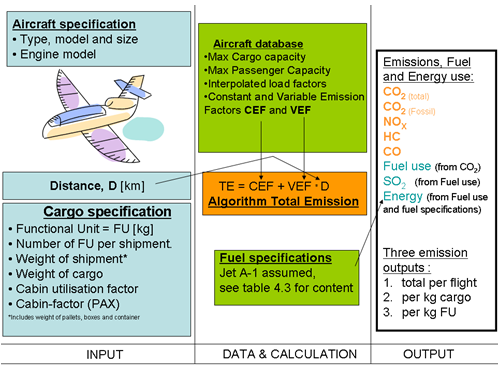Figure 1. A schematic view of the air transport calculations in NTM-calc.

The NTM emission database builds on computations made by FOI for NTM using data from the commercial software PIANO together with a FileMaker application  developed by FOI.

## Total emission calculation

Given that enough information is available the user can choose relevant emission figures from the NTM database where the emission factors are divided in two groups

• Constant Emission Factors (CEF) that corresponds to the high fuel usage during takeoff and landing.
• Variable Emission Factor (VEF) that is multiplied with the great circle distance in km.

This is a way to handle the other biased results by short respectively long flight and the importance of takeoff and landing in relation to cruising.

The linear equation describing the total emission is written as:

Equation 1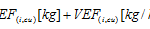Where

TEi = Total Emission of substance (i)

CEF(i,cu) = Constant Emission Factor for substance (i) at capacity utilisation (cu)

VEF(i,cu) = Variable Emission Factor for substance (i) at capacity utilisation (cu) and

D = Great Circle Distance between airports (GCD)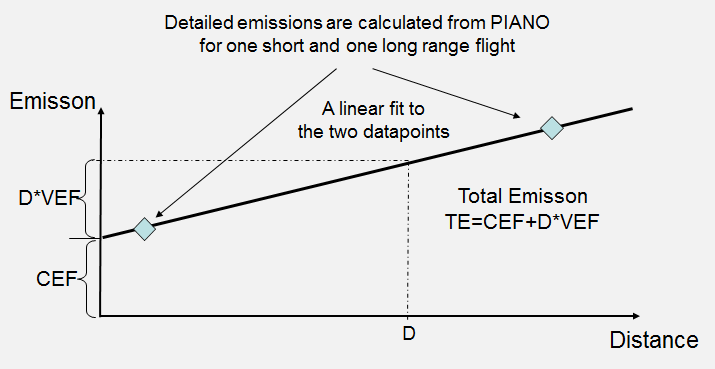Figure 2. Principals for emission calculation in NTM-calc.

## Emission factors CEF and VEF

CEF represents taxi out, start, climb to cruise, descend from cruise, landing and taxi in. The lateral flown distance during climb and decent are compensated for with a corresponding reduction in cruise fuel

Computations in the PIANO tool have been made using ICAO emission data  for the engines stated in appendix A,B and C and are valid for the use of Jet A-1 with the characteristics presented in Table 4-3.

Table 4-1below gives an example on CEFi and VEFi for CO2, NOx, HC, and CO for various load factors. Data for particle emissions is not included in this work since this data is missing in the emission reference being used. Calculation of energy and SOx is specified below in 4-3.

The full list of aircraft types and their corresponding set of data is available in NTMCalc.

 Aircraft Load factor (weight) Max Distance CO2 NOX HC CO CEF VEF CEF VEF CEF VEF CEF VEF [%] [km] [kg] [kg/km] [kg] [kg/km] [kg] [kg/km] [kg] [kg/km] B757-200SF 50 7051 4431 15.2 26.4 0.05 1.34 0.00 8.85 0.01 75 6712 4744 15.2 29.7 0.05 1.37 0.00 8.98 0.01 100 5184 5073 15.3 33.3 0.05 1.41 0.00 9.11 0.01 A310-300 F 50 9137 5628 20.7 49.5 0.09 2.36 0.00 11.45 0.01 75 9563 6159 18.3 55.2 0.07 2.41 0.00 11.62 0.01 100 7955 7033 18.0 65.2 0.07 2.50 0.00 11.92 0.01

Table 4‑1:  Example parameters for freight aircraft emission calculations

The emissions for a flight, including landing and take off (LTO) and cruising are calculated by inserting the distance flown into a linear equation, approximating the emission profile of respective plane type.

## Capacity utilisation (cu)

A separate linear fit is presented for at least three different levels of load factors; see Table 4-1. By using interpolation assuming linearity the user can calculate a emission factors for other load factors

Interpolation example:

CEF85% = CEF75% + (CEF100% – CEF75%) / (100%-75%) * (85%-75%)

 Freight factors Cabin factors [%] [%] Cargo aircraft 50, 75, 100 Passenger aircraft 40, 65, 90, 100 Belly freighters 50, 100 65, 90

Table 4‑2: Load factors used for computation of emission data

Load factors are based on specified maximum weight capacity for freight- and cabin factor, respectively, see appendix A, B and C. The cabin factor depends on the seating arrangement (normally configured for some comfort), which means that the maximum passenger load is usually lower than the cargo load would be in the corresponding freighter aircraft.

Note that for the belly freighters, data for four combinations of freight and cabin factors are presented, thus enabling linear interpolation for any pair of freight and cabin factors.

## Distance (D)

NTM base the trip distance on the methodology described by ICAO. The methodology uses the Great Circle Distance, GCD between two airports. The GCD defines the shortest distance between two points on the surface of a sphere. This distance can be calculated by using the geographical coordinates of the two point’s concerned (O&D, Origin & Destination). The coordinates for the airports involved can be obtained from the ICAO Location Indicators Database.

The great circle distance d between two points with coordinates {lat1, lon1} and {lat2, lon2} is given by:

Equation 2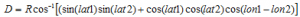A mathematically equivalent formula, which is less subject to rounding error for short distances, is:

Equation 3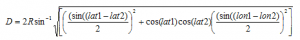Where R is the mean radius of the earth. R = 6371.01 km.

## Fuel characteristics

All planes are assumed to be operating on JetA-1 fuel with the following characteristics:

 FUEL DATA Unit Aviation fuel – Jet A Heat value [MJ/l] 34.9 [MJ/kg] 43.1 Energy content with renewable origin [MJ/l] 0 Energy content with fossil origin [MJ/l] 34.9 Energy content with nuclear origin [MJ/l] 0 TOTAL CO2 [kg/l] 2.6 FOSSIL CO2 [kg/l] 2.6 [kg/kg] 3.16 Carbon content [%-weight] Sulphur content [ppm-weight] 250 [%-weight] 0.025% SOx – Sulphur dioxide emission [g/l] 0.41 [g/kg] 0.50 Density7 (at +15º C) [kg/l] 0.8102

The data in Table 4-3 is suggested as a typical Jet A-1 specification, although the reference is old . Deviations from these values exist and ideally specific data for the fuel used for the specific transport should be obtained.

## Calculating fuel usage, SOx and energy utilization

The result of the CO2 calculation together with the fuel specification in Table 4-3 can be used for calculation of the following parameters:

• The fuel consumption, FC, is calculated by dividing the CO2 emission by the fuel-specific CO2 emission. (FC [kg]= CO2 [kg] / 3.16 [kg])
• The sulphur dioxide emission, SOx, is calculated by first multiplying FC by the sulphur content in order to find the amount of elementary sulphur emitted. This amount is then doubled in order to compensate for the increased weight of the sulphur dioxide molecule.
• The amount of energy utilised is calculated by multiplying the fuel consumption (FC) by the heat value stated in Table 4.3.

 LTO = Land and Take off cycle. The LTO cycle includes all movements below 3000 feet.

  International Civil Aviation Organization